# ISEE Lower Level Quantitative : How to find a proportion

## Example Questions

### Example Question #1 : How To Find A Proportion

A recipe calls for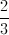of a cup of flour andof a cup of sugar.  How many cups of sugar and flour combined does the recipe call for?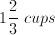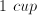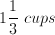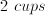Explanation:

Add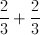Since both of these fractions have the same denominator, we just rewrite 3 on the bottom.  When you add fractions, then add straight across the top,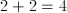.

This gives us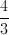.

We can turn this into a mixed number by dividing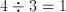with a remainder of 1.  We place the orginal one as our whole number, and the remainder over the 3 for the fraction,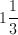.

### Example Question #1 : How To Find A Proportion

Which fraction is the greatest?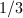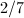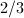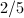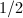Explanation:

As the denominator gets bigger, the fractions gets smaller, so when looking for the largest fraction, we are looking for one with the highest numerator and the lowest denominator.  In this case,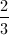has the largest numerator and the smallest denominator.

### Example Question #3 : How To Find A Proportion

Afoot tree casts a shadow offeet. How long is the shadow of a nearbyfoot tall building if the tree and its shadow are in the same ratio as the building and its shadow?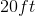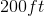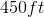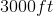Explanation:

To solve this, I need to set up a proportion, and then solve algebraically.feet, the height of the tree, is equivalent in our proportion tofeet, the building's height.feet, the tree's shadow, is equivalent in our proportion to, the building's shadow.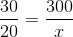Cross-multiply: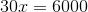Divide each side by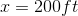### Example Question #1 : How To Find A Proportion

There are 18 tea bags in a box. Beth uses 2 tea bags, Brad uses 1 tea bag, and Joe uses 1 tea bag. What fraction of the tea bags now remain?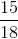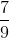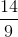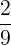Explanation:

If there are 18 tea bags in a box and Beth uses 2 tea bags, Brad uses 1 tea bag, and Joe uses 1 tea bag, then 14 tea bags will remain.

Given that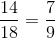, the correct answer is.

### Example Question #12 : Ratio And Proportion

If Katie's computer has 60% of its power left after being on for 2 hours, how many hours of battery power remain?Explanation:

If Katie's computer has 60% of its power left after being on for 2 hours, then that means 40% of the battery was used to power the 2 hours. X represents the total number of hours that the battery can last.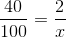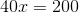Next, we divide each side of the equation by 40. This results in:Since 60% of 5 is equal to 3, the correct answer is 3.

### Example Question #6 : How To Find A Proportion

Marcus is 9 years old. He has been playing soccer for 3 years. If he plays soccer for a 4th year, for what proportion of his life will he have played soccer?Explanation:

If Marcus is 9 years old and has been playing soccer for 3 years, he will be 10 years old during his 4th year. He has therefore played soccer for 4 out of 10 years, or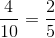of his life.

### Example Question #7 : How To Find A Proportion

Ralph watched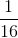of a movie that is 2 hours and 8 minutes long. How much of the movie did he watch (in minutes)?Explanation:

The first step is to convert the length of the movie from hours to minutes. A movie that is 2 hours and 8 minutes long is 128 minutes long.

128 divided by 16 is 8. Therefore, 8 minutes isof the movie.

### Example Question #8 : How To Find A Proportion

If 5 out of 20 students in a class have green eyes, what percentage of students have green eyes?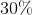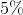Explanation:

Percentages are fractions out of 100, so the way to solve the problem is to have the denominator be 100. In order to do this we must multiply 20 by 5 in order to get 100.

If we multiply the denominator by 5, we must do the same to the numerator.

When we do this, we are left with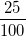which is 25%

### Example Question #9 : How To Find A Proportion

If Katie's computer has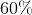of its power left after being on forhours, how many hours of battery power remain?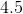Explanation:

If Katie's computer has 60% of its power left after being on for 2 hours, then that means 40% of the battery was used to power the 2 hours. X represents the total number of hours that the battery can last.Next, we divide each side of the equation by 40. This results in:Since 60% of 5 is equal to 3, the correct answer is 3.